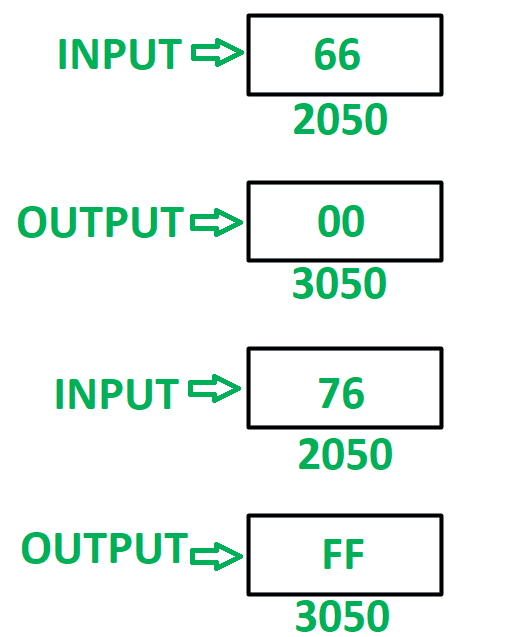GeeksforGeeks App
Open AppBrowser
Continue

# 8085 program to check whether both the nibbles of 8 bit number are equal or not

Problem – Write an assembly language program in 8085 microprocessor to check whether both the nibbles of 8 bit number are equal or not. If nibbles are equal then store 00 in memory location 3050 otherwise store FF in memory location 3050.

Example –Assumption – Number, to check for similar nibbles is stored at memory location 2050.

Algorithm –

1. Load the content of memory location 2050 in A.
2. Moves the content of A in B.
3. Mask the lower nibble and store it in register C.
4. Move the content of B in A.
5. Mask the higher order nibble and store it in A.
6. Reverse the content of A by using RLC instruction 4 times.
7. Compare the contents of A and C by help of CMP instruction. Update the flags of 8085.
8. Now store FF if ZF = 0 otherwise store 00 if ZF = 1.
9. Store the final result in memory location 3050.

Program –

2000LDA 2050A <- M
2003MOV B, AB <- A
2004ANI 0FA <- A (AND) 0F
2006MOV C, AC <- A
2007MOV A, BA <- B
2008ANI F0A <- A (AND) 0F
200ARLCRotate content of A left by one bit without carry
200BRLCRotate content of A left by one bit without carry
200CRLCRotate content of A left by one bit without carry
200DRLCRotate content of A left by one bit without carry
200ECMP CA – C
200FJZ 2018Jump if ZF = 1
2013MVI A, FFA <- FF
2018MVI A, 00A <- 00
201ASTA 3050M <- A
201DHLTEND

Explanation – Registers A, B, C are used for general purpose.

1. LDA 2050: load the content of memory location 2050 in accumulator A.
2. MOV B, A: moves the content of A in register B.
3. ANI 0F: performs AND operation in contents of A and 0F. Store the result in A.
4. MOV C, A: moves the content of A in register C.
5. MOV A, B: moves the content of B in A.
6. ANI F0: performs AND operation in contents of A and F0. Store the result in A.
7. RLC: rotates content of A left by one bit without carry. Use the instruction 4 times to reverse the number.
8. CMP C: compares the content of A and C. Update the flags of 8085 accordingly.
9. JZ 2018: jump to memory location 2018 if zero flag is set.
10. MVI A, FF: assign FF to A.